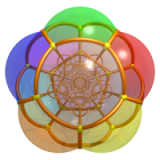# 具有超理想顶点的双曲蜂巢# 双曲动力系统的极限集与无限 Coxeter 群的极限根

$W$ 作为 Kleinian 群的极限集 $\Lambda(W)$ 有若干种不同的等价描述方式。

$x_0\in\mathbb{H}_3$ 是三维双曲空间中一点，$W(x_0)=\{w(x_0)\,|\, w\in W\}$$x_0$$W$ 作用下的轨道，则集合 $W(x_0)$ 的聚点都位于无穷远边界球面 $S^3$ 上。我们把 $W(x_0)$ 的所有聚点组成的集合叫做 $W$极限点，把极限点的闭包叫做 $W$极限集，记作 $\Lambda(W)$。可以证明 $\Lambda(W)$ 的定义不依赖于 $x_0$ 的选取，并且 $\Lambda(W)$ 是理想球面上在 $W$ 作用下不变的最小非空闭集。

$W$ 作为无限 Coxeter 群，其极限根的概念则是最近几年才提出。完整的描述极限根的概念需要颇为一番周折，我这里简单尝试介绍一下，恐怕不见得说得十分明白，读者最好还是参考论文如 3 4 等。

$\Delta = \{\alpha,\beta,\gamma,\delta\}$$W$ 的单根集，$\Phi$$(W,\Delta)$ 的根系，$\Phi^+$ 是其中的正根。

# 前面的插图是怎么画出来的?

Roice 的代码在这里，我正在考虑未来有时间用 C++ 把这个项目的精彩部分用 Coxeter 群的有限状态机的方法实现一遍。

# 彩蛋1. Visualizing Hyperbolic Honeycombs, Roice Nelson, Henry Segerman.↩︎

2. 这里需要证明每个正根 $\rho$ 所在的方向确实与 $V_1$ 有交点，见脚注 4 中的文献。↩︎